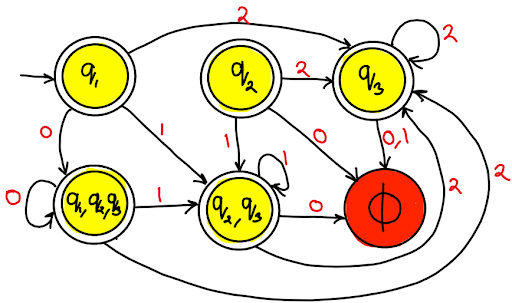## NFA to DFA using Indirect method

Step 01 : First convert NFA with ∈ moves to NFA without ∈ moves.
Step 01 :Then convert NFA without ∈ to the DFA.

Let's take an example,

We have a NFA with epsilon moves, now convert it to the NFA without epsilon moves.NFA with epsilon moves

First convert above NFA to without epsilon moves,

Find ∈-closure of (q0), (q1) and (q2).
1. ∈-closure of (q0) = {q0, q1, q2}
2. ∈-closure of (q1) = {q1, q2}
3. ∈-closure of (q2) = {q2}

 State 0 1 2 ->q1 {q1,q2,q3} {q2,q3} {q3} q2 φ {q2,q3} {q3} q3 φ φ {q3}

From the above transition table, draw the transition diagram,NFA without  epsilon

From the question diagram, it is clear that only with ∈ input q1 and q2 state can reach to the final state.
So, now without ∈ input, q1 and q2 is also treated as final states.
As shown in diagram beabove.

Now, NFA without epsilon to DFA

Using Subset construction method.

Again I am going to use information from this transition table.

 State 0 1 2 ->q1 {q1,q2,q3} {q2,q3} {q3} q2 φ {q2,q3} {q3} q3 φ φ {q3}

Subsets are,
1. {q1}
2. {q2}
3. {q3}
4. {q1,q2,q3}
5. {q2,q3}
Treat each subset as state in DFA.
If there is no transition use φ symbol.

 State Input = 0 Input = 1 Input = 2 {q1} {q1,q2,q3} {q2,q3} {q3} {q2} φ {q2,q3} {q3} {q3} φ φ {q3} {q1,q2,q3} {q1,q2,q3} {q2,q3} {q3} {q2,q3} φ {q2,q3} {q3} φ φ φ φ

In NFA withoud epsilon, q1, q2, q3 was final states. In subset {q1,q2,q3} and {q2,q3} final states exist.
So these subsets will also treated as final states.DFA from NFA

Now from the transition table of above DFA,

 State Input = 0 Input = 1 Input = 2 {q1} {q1,q2,q3} {q2,q3} {q3} {q2} φ {q2,q3} {q3} {q3} φ φ {q3} {q1,q2,q3} {q1,q2,q3} {q2,q3} {q3} {q2,q3} φ {q2,q3} {q3} φ φ φ φ

We find state {q1,q2,q3} is equivalent to state {q1}, so replce state {q1,q2,q3} by state {q1}.
Simillary, replace state {q2,q3} by state {q2}.

So, new DFA transition table become,

 State Input = 0 Input = 1 Input = 2 {q1} {q1} {q2} {q3} {q2} φ {q2} {q3} {q3} φ φ {q3} φ φ φ φ

And DFA transition diagram,

Share:
ACA: ADVANCED COMPUTER ARCHITECTURE EasyExamNotes.com covered following topics in these notes.
Discrete Strucutre ↓ 👆
Discrete Structure EasyExamNotes.com covered following topics in these notes.
A list of Video lectures
Information Storage Management ↓ 👆
Network & Web Security ↓ 👆
Web Engineering ↓ 👆
Web Engineering EasyExamNotes.com covered following topics in these notes.
Cloud Computing ↓ 👆

Cloud Computing:

EasyExamNotes.com covered following topics in Cloud Computing.

1. Buyya, Selvi ,” Mastering Cloud Computing “,TMH Pub
2. Krutz , Vines, “Cloud Security “ , Wiley Pub
3. Velte, “Cloud Computing- A Practical Approach” ,TMH Pub
4. Sosinsky, “ Cloud Computing” , Wiley Pub
Python Programming ↓ 👆
Principles of Programming Languages ↓ 👆
Principles of Programming Languages
EasyExamNotes.com covered following topics in these notes.

#### Language Evaluation Criteria Influences on Language Design  Language Categories Programming Paradigms Compilation Virtual Machines  Programming Environments Issues in Language Translation Parse Tree  Pointer and Reference type Concept of Binding Type Checking Strong typing  Sequence control with Expression Subprograms Fundamentals of sub-programs Scope and lifetime of variable Static and dynamic scope Design issues of subprogram and operations Local referencing environments Parameter passing methods Overloaded sub-programs Generic sub-programs  Design issues for functions  Co routines  Abstract Data types Abstraction and encapsulation Static and Stack-Based Storage management Garbage Collection OOP in C++ OOP in Java  OOP in C#  OOP in PHP Concurrency Semaphores  Monitors Message passing Java threads  C# threads  Exception handling  Exceptions Exception Propagation  Exception handler in C++  Exception handler in Java Introduction and overview of Logic programming Basic elements of Prolog Application of Logic programming Functional programming languages Introduction to 4GL  Practicals: Memory Implementation of 2D Array.  Memory Implementation of 3D Array.  Implementation of pointers in C++.  Write a program in Java to implement exception handling. Write a program in C++ to implement call by value parameter passing Method. Write a program in C++ to implement call by reference parameter passing Method.  Write a program in Java to implement concurrent execution of a job using threads. Implement Inheritance in C#. Implement Encapsulation in C#. Implement static/compiletime Polymorphism in C#. Implement dynamic/runtime Polymorphism in C#. Previous years solved papers: PPL|RGPV|May 2018  PPL|RGPV|June 2017  A list of Video lectures Click here References: Sebesta,”Concept of programming Language”, Pearson Edu  Louden, “Programming Languages: Principles & Practices” , Cengage Learning  Tucker, “Programming Languages: Principles and paradigms “, Tata McGraw –Hill.  E Horowitz, "Programming Languages", 2nd Edition, Addison Wesley

Computer Organization and Architecture ↓ 👆

### Computer Organization and Architecture

#### References:

1. William stalling ,“Computer Architecture and Organization” PHI
2. Morris Mano , “Computer System Organization ”PHI

Theory of Computation ↓ 👆
TOC EasyExamNotes.com covered following topics in these notes.
Computer Network ↓ 👆
Computer Network

EasyExamNotes.com covered following topics in these notes.
References:
1. Andrew S. Tanenbaum, David J. Wetherall, “Computer Networks” Pearson Education.
2. Douglas E Comer, “Internetworking with TCP/IP Principles, Protocols, And Architecture",Pearson Education
3. KavehPahlavan, Prashant Krishnamurthy, “Networking Fundamentals”, Wiley Publication.
4. Ying-Dar Lin, Ren-Hung Hwang, Fred Baker, “Computer Networks: An Open Source Approach”, McGraw Hill.
R Programming ↓ 👆

### R Programming

EasyExamNotes.com covered following topics in these notes.

Management Information System ↓ 👆